BSP-Net

## 参考论文

BSP-Net: Generating Compact Meshes via Binary Space Partitioning

## Introduction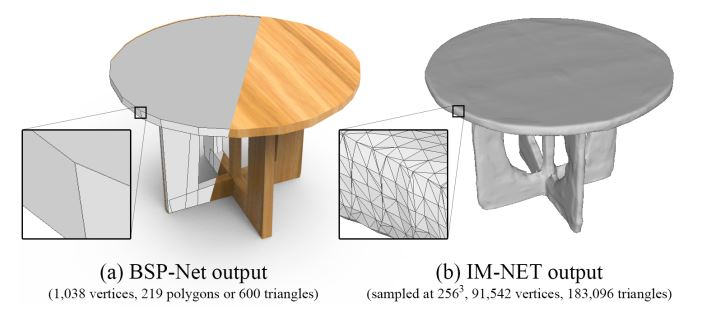## Background

### Bianry Space Partitioning(BSP-Tree)

#### 概念

BSP树是一种数据的组合形式，构建的过程从某种类型的数据开始，将其分成两个部分，再将这两个部分继续分割，直到得到最小的单元。

#### BSP Tree in Computer Graphics

BSP Tree起初用于加快渲染器的渲染速度：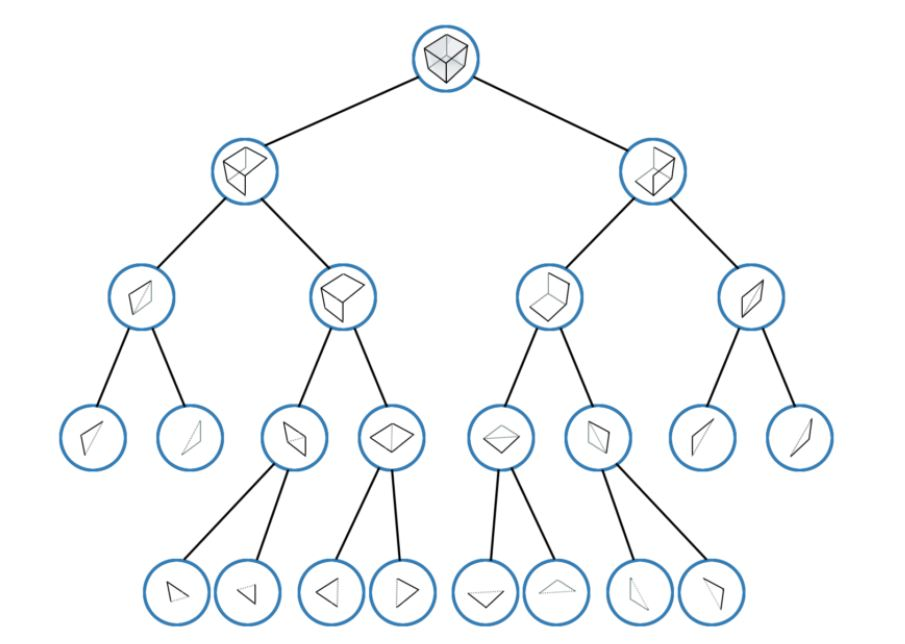### Construct Solid Geometry(CSG)

CSG是通过一组基元以及布尔运算来表示一个物体的方法，运算包括求并集、交集以及相减等。在这片论文中，在获得了凸多面体集合后，采用并集的方式得到显式的多面体网格模型。

## Method

BSP-Net学习了一个Implicit field，给定n个点的坐标，网络输出对应的值表示该点是否位于模型内。

1. 一个含有p个平面等式的集合P，每个平面将空间分割成了两个部分。

2. 矩阵$T_{p \times c}$将上一步中的平面进行聚合，得到c个凸多面体作为三维模型的基元（primitives）/部分（parts）。

3. 最后，通过对上述基元的组合，得到单一的表示输出模型的implicit field。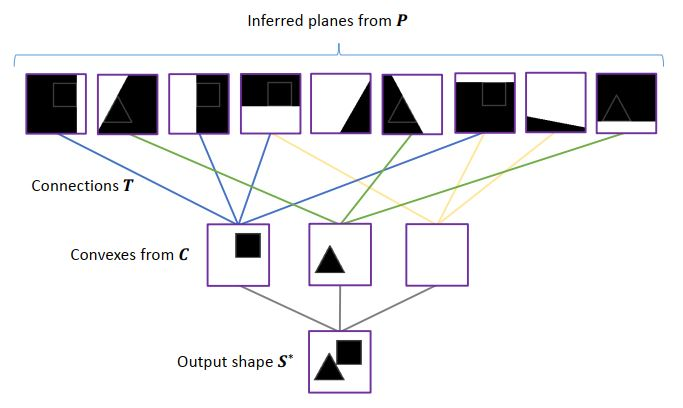### Archetecture of BSP-Net

BSP-Net的网络结构如下图所示：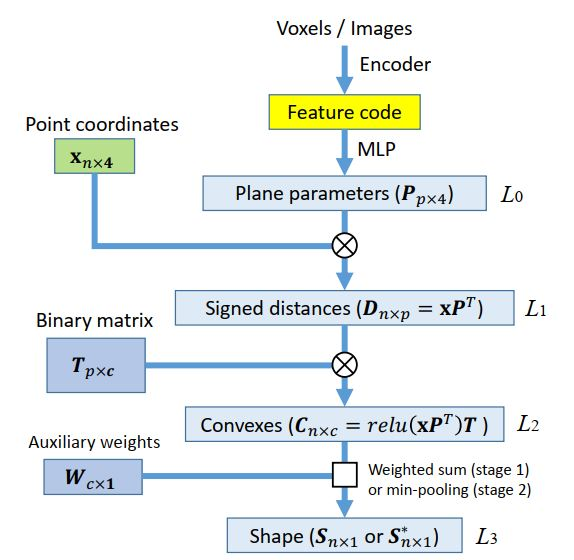#### Layer 2: hyperplane grouping

$T_{p \times c}$为二值矩阵，用于选取平面将其组合为c个凸多面体，输出为矩阵$C_{n \times c}$表示凸多面体集合，在网络中，采用加和的方式将选定的平面聚合在一起：

$C^+_j(x) = \sum_irelu(D_i)Tij \left\{ \begin{array}{ll} = 0 & inside \\ > 0 & outside \end{array} \right.$

#### Layer 3: shape assembly

$S^*(x) = \min_{j}(C^+_j(x)) \left\{ \begin{array}{ll} = 0 & inside \\ > 0 & outside \end{array} \right.$

$S^+(x) = \left[ \sum_jW_j[1 - C^+_j(x)]_{[0, 1]} \right]_{[0,1]} \left\{ \begin{array}{ll} =1 & \approx in \\ [0,1) & \approx out \end{array} \right.$

### Two-stage training

$S^+(x)$相较于$S^*(x)$虽然仅是一种估计，但是更易训练。在训练过程中，作者分为了两个步骤：

1. 连续阶段：将所有的权重看作连续的值，通过$S^+(x)$计算一个大致的模型。

2. 离散阶段：将权值离散化，通过在$S^*(x)$上的微调得到更加精确的结果。

#### Training Stage 1 - Continuous

$\mathop{\arg\min}_{\omega, T, W} \mathcal{L}^+_{rec} + \mathcal{L}^+_{T} + \mathcal{L}^+_{W}$

$\mathcal{L}^+_{rec} = \mathbb{E}_{x \sim G}\left[ (S^+(x) - F(x|G))^2 \right]$

$\mathcal{L}^+_T = \sum_{t \in T}max(-t, 0) + \sum_{t \in T}max(t-1, 0)$

$\mathcal{L}^+_W = \sum_{j}|W_j - 1|$

#### Training Stage 2 - Discrete

$\mathop{\arg\min}_{\omega} \mathcal{L}^*_{recon} + \mathcal{L}^*_{overlap}$

$\mathcal{L}^*_{recon} = \mathbb{E}_{x \sim G}[F(x|G) \cdot max(S^*(x), 0)] + \mathbb{E}_{x \sim G}[(1-F(x|G))\cdot(1-min(S^*(x),1))]$

$\mathcal{L}^*_{overlap} = -\mathbb{E}_{x \sim G}[M(x)S^*(x)]$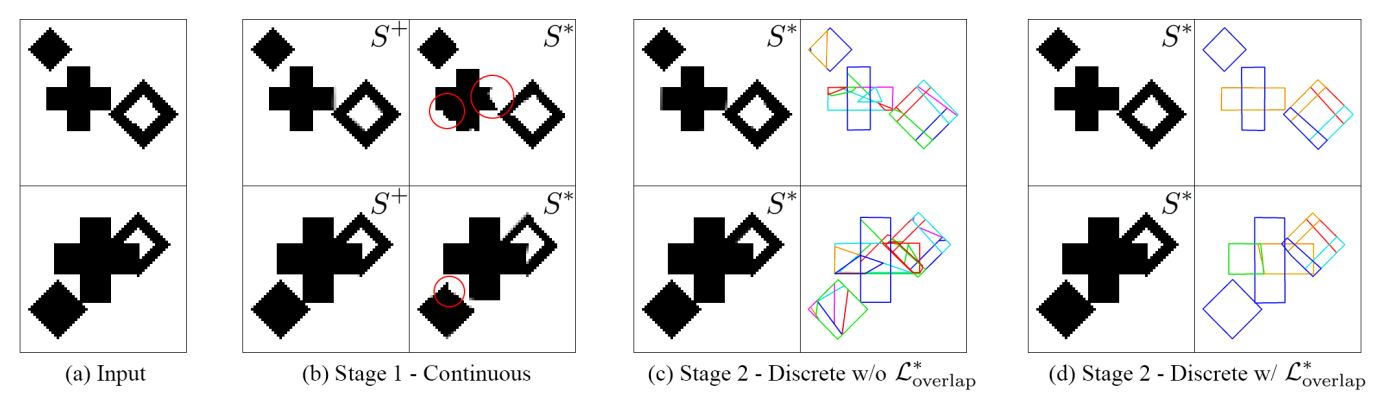## Conclusion

• BSP-Net提出了一种利用BSP-Tree来表示三维模型的方法，对于输入的feature code，通过BSP-Net得到表示三维模型的BSP-Tree，再在树的基础上利用CSG建立多边形网格用于表示三维模型。在训练过程中，不需要多边形网格模型作为ground true参与训练，因此，网络的训练时self-supervised的，但依然需要依靠$F(x|G)$

• 对于BSP-Tree这一种三维模型的表示方法，它是可被训练的，因为对于几何特征的编码采用了隐式函数的方法；而且，BSP-Tree的表示方法是可解释的，因为它的输出为凸多面体的集合。

• 这一表示方法可用于对三维模型的自编码、语义分割以及Single view reconstruction(SVR)等应用领域。

• 但是，BSP-Net对于凹面形状的处理是将其分割成多个凸多面体，这并不是一种特别好的处理方式。除此之外，BSP-Net的训练速度相对较慢。